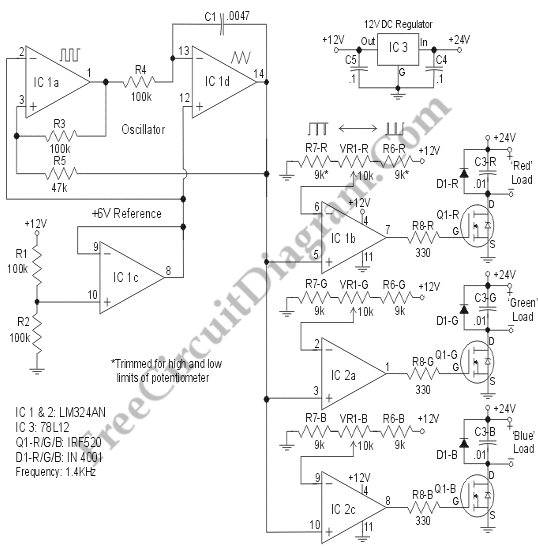# PWM RGB ControllerThe design of a Pulse Width Modulated Red-Green-Blue controller will be explained here. This design is efficient because this design uses multiple strings of six LEDs with a 24V DC source. This design is sufficient for a sizeable light because a total current of 2 Amps will supply 600 LEDs per color. The circuit below makes use of a remarkable device, a power MOSFET (Metal Oxide Silicon Field Effect Transistor).MOSFET here acts like an electronic relay that can switch from OFF to ON in a very short time and requires a miniscule control signal. We can switch up to 10 A DC in a few microsecond on type we use here. This design use IC1a and IC1b form an oscillator where only the triangular waveform. A reference voltage of 6 V DC is generated by IC1c. The triangular waveform is applied to three comparators (IC1b, IC2a,and IC2c). A variable voltage is applied to the regulators inverting input. Thus, the comparators will generate a pulse with a variable pulse width as the output. This drives the MOSFET switch. To step sown the raw 24V DC voltage to 12V DC for the the operational amplifiers, IC3 is used. The diodes D1x suppress reverse voltages that could result from long wiring. To minimize the interference, the capacitors C3x take the edge off the output pulse. [Circuit’s schematic diagram source: http://jeuch.us/index.html]